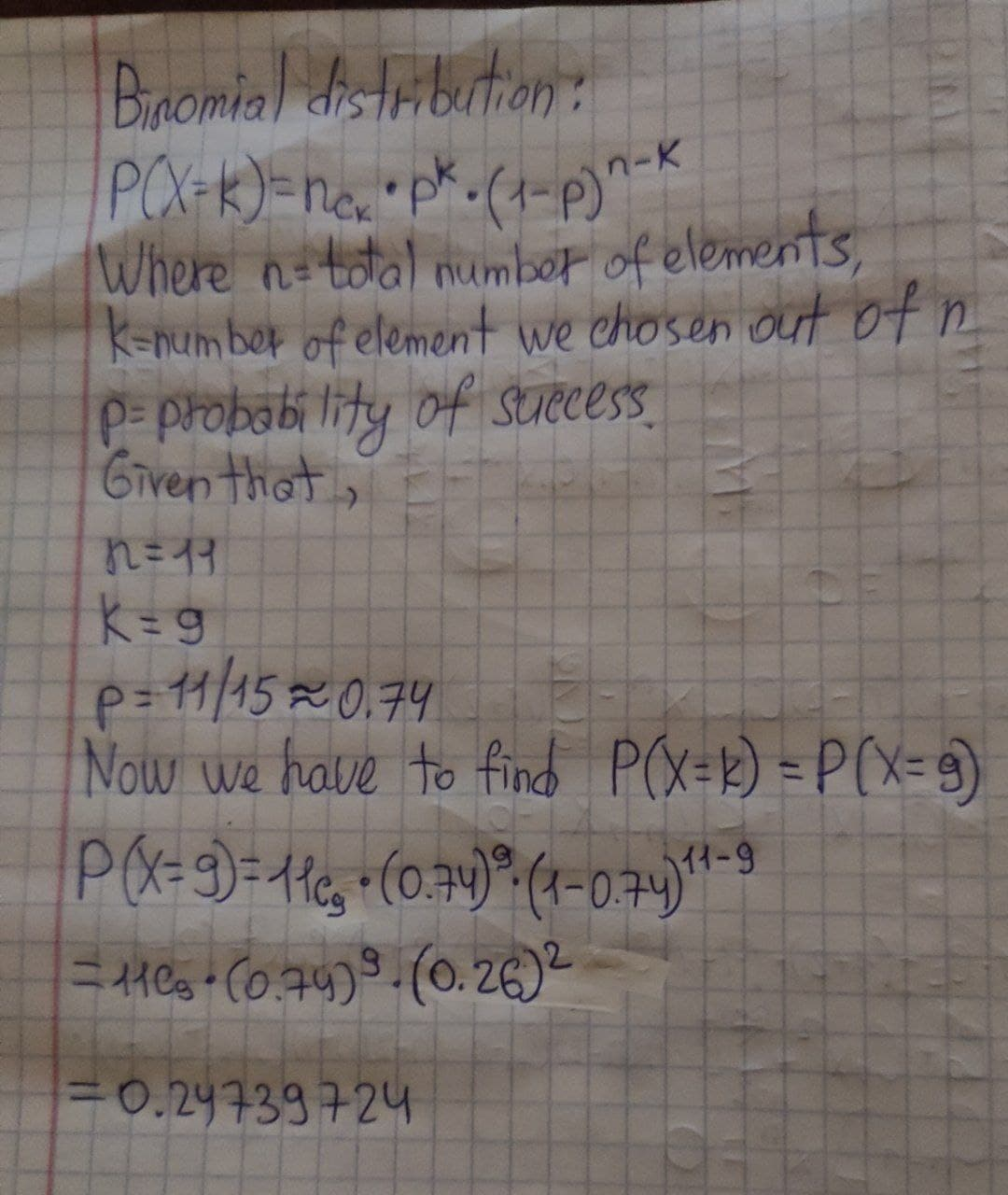2022-02-26

Assume that a procedure yields a binomial distribution with a trial repeated n = 11 times. Use the binomial probability formula to find the probability of k = 9 successes given the probability p = 11/15 of success on a single trial.alenahelenash